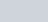The angles of a quadrilateral are in A.P. whose common
Question:

The angles of a quadrilateral are in A.P. whose common difference is 10°. Find the numbers.

Solution:

Here, we are given that the angles of a quadrilateral are in A.P, such that the common difference is 10°.

So, let us take the angles as $a-d, a, a+d, a+2 d$

Now, we know that the sum of all angles of a quadrilateral is 360°. So, we get,

$(a-d)+(a)+(a+d)+(a+2 d)=360$

$a-d+a+a+d+a+2 d=360$

$4 a+2(10)=360$

$4 a=360-20$

On further simplifying for a, we get,

$a=\frac{340}{4}$

$a=85$

So, the first angle is given by,

$a-d=85-10$

$=75^{\prime}$

Second angle is given by,Third angle is given by,

$a+d=85+10$

$=95^{\circ}$

Fourth angle is given by,

$a+2 d=85+(2)(10)$

$=85+20$

$=105$

Therefore, the four angles of the quadrilateral are $75^{\circ}, 85^{\circ}, 95^{\circ}, 105^{\circ}$.